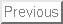Next: About this document ... Up: My Home Page

Rectilinear Grids

Rectilinear grids are typically defined by regularly spaced horizontal and vertical lines.

There are three important components of the planar grid: the vertices, the edges, and the cell interiors. Sometimes we are interested in the interiors of the cells, as in geometric applications where each cell describes a region in space. Sometimes we are interested in the vertices of the grid, such as in addressing the pieces on a chessboard. Sometimes we are interested in the edges of the grid, such as when finding routes to travel in a city where buildings occupy the interior of the cells.

Vertices in planar grids each touch four edges and the interiors of four cells, except for vertices on the boundaries. Vertices in 3D grids touch on six edges and eight cells. In-dimensions, each vertex touches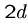edges andcells. Cells in a planar grid each touch eight faces, four diagonally through vertices and four through edges. Cells in a 3D grid each touch 26 other cells, sharing a face with 6 of them, an edge with 12 of them, and just a vertex with the other 8.

Traversal

It is often necessary to traverse all the cells of an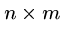rectilinear grid. Any such traversal can be thought of as a mapping from each of the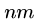ordered pairs to a unique integer from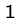to.

The most important traversal methods are --

• Row Major - Here we slice the matrix between rows, so the firstelements visited belong to the first row, the second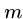elements to the second row, and so forth.

• Column Major - Here we slice the matrix between columns, so the first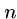elements belong to the first column, the secondelements to the second column, and so forth. This can be done by interchanging the order of the nested loops from row-major ordering. Knowing whether your compiler uses row-major or column-major ordering for matrices is important when optimizing for cache performance and when attempting certain pointer-arithmetic operations.

• Diagonal Order - Here we march up and down diagonals. Note that angrid hasdiagonals, each with a variable number of elements. This is a trickier task than it appears at first glance.

Dual Graphs and Representations

Two-dimensional arrays are the natural choice to represent planar rectilinear grids. We can let m[i][j] denote either the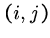th vertex or theth face, depending on which we are interested in. The four neighbors of any cell follow by adding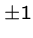to either of the coordinates.

A useful concept in thinking about problems on planar subdivisions is that of the dual graph, which has one vertex for each region in the subdivision, and edges between the vertices of any two regions which are neighbors of each other.

Observe that the dual graphs of both rectangular and hexagonal lattices are slightly smaller rectangular and hexagonal lattices. This is why whatever structure we use to represent vertex connectivities can also be used to represent face connectivities.

An adjacency representation is the natural way to represent an edge-weighted rectilinear grid. This might be most easily done by creating a three-dimensional array m[i][j][d], where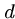ranges over four values (north, east, south, and west) which denote the edge directions from point.

Triangular Lattices

Triangular lattices are constructed from three sets of equally spaced lines, consisting of a horizontal row'' axis, a column'' axis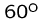from horizontal, and a diagonal'' axisfrom horizontal.

Vertices of this lattice are formed by the intersection of three axis lines, so each face of the lattice is an equilateral triangle. Each vertex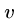is connected to six others, those immediately above and belowon each of the three axes.

To identify the proper neighbors of each vertex requires keeping track of two types of coordinate systems:

• Triangular/Hexagonal Coordinates - Here, one vertex is designated as the origin of the grid, point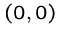. We must assign the coordinates such that the logical neighbors of each vertex are easily obtainable. In a standard rectilinear coordinate system, the four neighbors of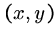follow by addingto either the row or column coordinates.

Although the intersection of three lines defines each grid vertex, in fact the row and column dimensions to specify location.

A vertexlies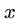rows above the origin, and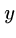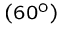-columns to the right of the origin. The neighbors of a vertexcan be found by adding the following pairs to the coordinates of, in counterclockwise order: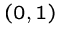,,,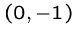,, and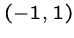.

• Geometrical Coordinates - The vertices of a regular triangular grid occur in half-staggered rows.

Assume that each lattice point is a distancefrom its six nearest neighbors, and that pointin triangular coordinates in fact lies at geometric point. Then triangular-coordinate point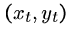must lie at geometric point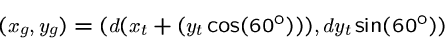by simple trigonometry, where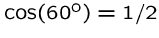and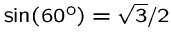,

Hexagonal Lattices

Deleting every other vertex from a triangular lattice leaves us with a hexagonal lattice. Now the faces of the lattice are regular hexagons, and each hexagon is adjacent to six other hexagons. The vertices of the lattice now have degree 3, because this lattice is the dual graph of the triangular lattice.

Hexagonal lattices have many interesting and useful properties, primarily because hexagons are rounder'' than squares.

To convert between triangular/hexagonal coordinates and geometrical coordinates, we assume that the origin of both systems is the center of a disk at.

The hexagonal coordinate (xh,yh) refers to the center of the disk on the horizontal row xh and diagonal column yh. The geometric coordinate of such a point is a function of the radius of the disk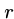, half that of the diameterdescribed in the previous section:


hex_to_geo(int xh, int yh, double r, double *xg, double *yg)
{
*yg = (2.0 * r) * xh * (sqrt(3)/2.0);
*xg = (2.0 * r) * xh * (1.0/2.0) + (2.0 * r) * yh;
}

geo_to_hex(double xg, double yg, double r, double *xh, double *yh)
{
*xh = (2.0/sqrt(3)) * yg / (2.0 * r);
*yh = (xg - (2.0 * r) * (*xh) * (1.0/2.0) ) / (2.0 * r);
}


The row-column nature of the hexagonal coordinate system implies a very useful property, namely that we can efficiently store a patch of hexagons in a matrix m[row][column]. By using the index offsets described for triangular grids, we can easily find the six neighbors of each hexagon.

There is a problem, however. Under the hexagonal coordinate system, the set of hexagons defined by coordinates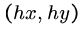, where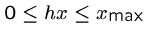and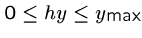, forms a diamond-shaped patch, not a conventional axis-oriented rectangle. However, for many applications we are interested in rectangles instead of diamonds.

To solve this problem, we define array coordinates so that (ax,ay) refers to the position in an axis-oriented rectangle withas the lower-left-hand point in the matrix:


array_to_hex(int xa, int ya, int *xh, int *yh)
{
*xh = xa;
*yh = ya - xa + ceil(xa/2.0);
}

hex_to_array(int xh, int yh, int *xa, int *ya)
{
*xa = xh;
*ya = yh + xh - ceil(xh/2.0);
}


Longitude and Latitude

A particularly important coordinate grid is the system of longitude and latitude which uniquely positions every location on the surface of the Earth.

The lines that run east-west, parallel to the equator, are called lines of latitude. The equator has a latitude of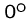, while the north and south poles have latitudes of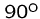North andSouth, respectively.

The lines that run north-south are called lines of longitude or meridians. The prime meridian passes through Greenwich, England, and has longitude, with the entire range of longitudes spanning fromWest toEast.

Every location on the surface of the Earth is described by the intersection of a latitude line and a longitude line. For example, the center of the universe (Manhattan) lies at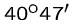North and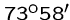West.

A great circle is a circular cross-section of a sphere which passes through the center of the sphere. The shortest distance between pointsandturns out to be the arc length betweenandon the unique great circle which passes throughand.

Denote the position of point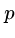by its longitude-latitude coordinates,, where all angles are measured in radians. Then the great-circle distance between pointsand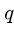is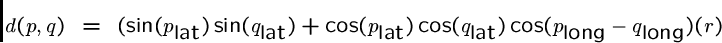Assigned Problems

111202 (The Monocycle) - Find the fastest way to move across a grid with blocked cells, where (1) you pay for each move and turn, and (2) you must start and end on the same color of a 5-color wheel. What is the right graph to capture the full structure?

111203 (Star) - Given row, column, and diagonal sums across a chinese checker board, what are the maximum and minimum possible sums of all the squares on the board?

111205 (Robbery) - Given observations of the form the robber isn't in a given rectangle at a given time'', identify where the robber might have been when.

111207 (Dermuba Triangle) - Find the shortest path (distance) between two houses on a triangular grid.Next: About this document ... Up: My Home Page
Steve Skiena
2003-05-22